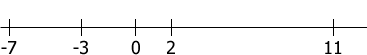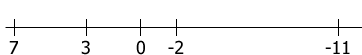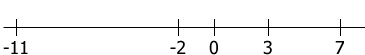SEARCH HOMEMath Central Quandaries & QueriesQuestion from Bryauna, a student: Why do you change the signs in inequalities?!Hi,

You change the direction of an inequality if you multiply both sides by the same negative number. Why is this?

Think about the number line and the way we draw it. We put the negative numbers on the left and the positive numbers on the right, or more generally if two numbers are on the line then the one to the right is larger than the one to the left. Here is a piece of the number line with a few points on it.Diagram 1

Now change the sign of all the numbers, or you might say you multiply each number by $-1.$ This givesDiagram 2

But this isn't correct, we want the negative numbers on the left and the positive numbers on the right. To fix the diagram we need to turn it around to obtainDiagram 3

From the first diagram we can see that $2 < 11$ and $-7 < 2$ and from the third diagram $-11 < -2$ and $-2 < 7.$

Multiplying by a negative number turns the number line around.

I hope this helps,
PennyMath Central is supported by the University of Regina and The Pacific Institute for the Mathematical Sciences.